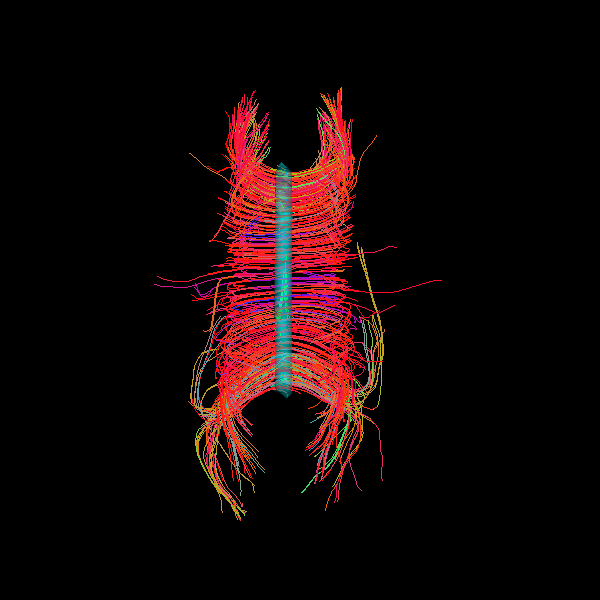# Visualization of ROI Surface Rendered with Streamlines#

Here is a simple tutorial following the probabilistic CSA Tracking Example in which we generate a dataset of streamlines from a corpus callosum ROI, and then display them with the seed ROI rendered in 3D with 50% transparency.

```from dipy.data import default_sphere, read_stanford_labels
from dipy.direction import peaks_from_model
from dipy.reconst.shm import CsaOdfModel

try:
from dipy.tracking.local import LocalTracking
from dipy.tracking.local import (
ThresholdTissueClassifier as ThresholdStoppingCriterion,
)
except ImportError:
from dipy.tracking.stopping_criterion import ThresholdStoppingCriterion
from dipy.tracking.local_tracking import LocalTracking

from dipy.tracking import utils
from dipy.tracking.streamline import Streamlines

from fury import actor, window
from fury.colormap import line_colors
```

First, we need to generate some streamlines. For a more complete description of these steps, please refer to the CSA Probabilistic Tracking Tutorial.

```hardi_img, gtab, labels_img = read_stanford_labels()
data = hardi_img.get_fdata()
labels = labels_img.get_fdata()
affine = hardi_img.affine

white_matter = (labels == 1) | (labels == 2)

csa_model = CsaOdfModel(gtab, sh_order=6)
csa_peaks = peaks_from_model(
csa_model,
data,
default_sphere,
relative_peak_threshold=0.8,
min_separation_angle=45,
)

classifier = ThresholdStoppingCriterion(csa_peaks.gfa, 0.25)

# Initialization of LocalTracking. The computation happens in the next step.
streamlines = LocalTracking(csa_peaks, classifier, seeds, affine, step_size=2)

# Compute streamlines and store as a list.
streamlines = Streamlines(streamlines)
```

We will create a streamline actor from the streamlines.

```streamlines_actor = actor.line(streamlines, line_colors(streamlines))
```

Next, we create a surface actor from the corpus callosum seed ROI. We provide the ROI data, the affine, the color in [R,G,B], and the opacity as a decimal between zero and one. Here, we set the color as blue/green with 50% opacity.

```surface_opacity = 0.5
surface_color = [0, 1, 1]

seedroi_actor = actor.contour_from_roi(
)
```

Next, we initialize a ‘’Scene’’ object and add both actors to the rendering.

```scene = window.Scene()
```

If you uncomment the following line, the rendering will pop up in an interactive window.

```interactive = False
if interactive:
window.show(scene)

# scene.zoom(1.5)
# scene.reset_clipping_range()

window.record(scene, out_path='contour_from_roi_tutorial.png', size=(600, 600))
```Total running time of the script: ( 0 minutes 19.063 seconds)

Gallery generated by Sphinx-Gallery